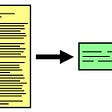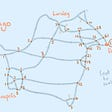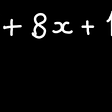# 3 Basic Concepts in Linear Algebra

a beginner’s primer on vectors, dot products, and linear independence

If you don’t have much training in linear algebra it’s hard crack open a textbook and identify the most important concepts. You can get lost in all the examples. This post describes three of the most important concepts in linear algebra along with some interesting examples and counter examples.

1. Vectors (as algebraic and geometric objects)
2. Dot products of vectors with a geometric interpretation
3. Linear independence/dependence of sets of vectors

After reading this you should have a good feel for the fundamentals and the motivation to learn more. To keep things simple, for this post we will only consider vectors over the real numbers. However many of the concepts will carry over to vectors over other fields.

## Vectors

A vector is an ordered list of numbers. Let’s start by dealing only with vectors of real numbers. For example (3, 1, 1) is a vector with the first entree equal to 3, the second 2, and the third 1.

Vectors correspond to points in space. We usually represent them as arrows from the origin of a graph to a point. In the graph below the red arrow corresponds to the vector (3,1,1) because the coordinates of the endpoint is x = 3, y = 1, and z = 1. The blue arrow is the vector (0, 2, 2) since the coordinates of its end point is x = 0, y = 2, and z = 2. These vectors are 3-dimensional because they have have three entries.

Vectors can be 1-dimensional, 2-dimensional, 3-dimensional, or any number of dimensions. But once we get above 3-dimensions it becomes extremely inconvenient to visualize them on a graph.

## The zero vector

In each dimension, there is a unique vector called the zero-vector. It is a vector with all zero entries. In two dimensions we have (0,0), in three dimensions we have (0,0,0), four dimensions (0,0,0,0) and so on. When the dimension is understood by context, we symbolize the zero vector as 0.

## Standard basis vectors

Standard basis vectors have a 1 in exactly one of their entries and 0 for every other entry. For example (0,0,1,0,0,0) is a 6-dimensional standard basis vector. As long as the dimension is understood, we symbolize standard basis vectors with bold e with a subscript indicating the position of the 1 entry.

In two dimension there are two Standard basis vectors, in three dimensions there are three, and so on.

## Vector addition, scalar multiplication, and dot product

We can add vectors to get new vectors. The way we add is by adding their respective components. We can only add vectors that have the same dimension. For example (3,1,1) + (0,2,2) = (3,3,3).

Adding vectors has a geometric interpretation. The sum of two vectors is the vector you get when you translate one vector so that its tail is connected to the head of the other vector. As long as you don’t change the direction of the vector you are translating we will get the sum of the two vectors. It doesn’t matter which vector you choose to translate.

The dotted orange vector is the sum of the blue and red vectors. It has coordinates (3,3,3) just as we expected.

## Scalar multiplication

We can multiply a vector by a regular number to get another vector. This is called scalar multiplication. We do that by multiplying each entry of the vector by the number. For example 2(3,1,1) = (6,2,2). Scalar multiplication does not change the direction of a vector (unless we multiply by zero). It just changes the length of the vector.

Fact: Let’s say we have a vector, v. If we add v to -v we get the zero vector.

Fact: Every vector can be written as the sum of standard basic vectors multiplied by scalars as we can see in the three dimensional example below.

## The dot product

The dot product of two vectors is the sum of the products of each of their corresponding entries. So the result of a dot product is not a vector — it’s a scalar. For example.

In general, for n-dimensional vectors u and v,

It’s easy to see that the distributive property works for the dot product.

Fact: Any vector multiplied by 0 is the zero vector.

## Length of a vector

Given a vector of real numbers we can find the length of a vector using the Pythagorean Theorem. That is we take the square root of the sum of the squares of the entries. We use double vertical bars to indicate length. Let’s try (3,1,1) for example,

Fact: All standard basis vectors have length 1.

We can also write the length of a vector with the dot product.

Fact: A vector of length 0 is the zero vector. This is easy to see.

## Geometric representation of the dot product

Just like vector addition has a geometric interpretation, so does the dot product. The dot product of two vectors u and v is the products of their lengths multiplied by the cosine of the angle between them.

To see why this is true consider the product of the vector (u-v) with itself. This is the square of the length of (u-v). Use the distributive property to expand.

The vectors u, v, and u-v form a triangle. Apply the law of cosines to the lengths to get the following

where θ is the angle between the two vectors u and v. Use these two equations to solve for the dot product of u and v. This gives us a geometric form form the dot product. If θ is the angle between the two vectors u and v then,

Vectors that have a dot product of zero are said to be orthogonal because the angle between them is a right angle.

## Rows and columns

So far we have been writing vectors horizontally, as rows. Depending on the context we may also write vectors as columns.

Until we start to discuss matrices it doesn’t matter if we use rows or columns to characterize vectors.

## Linear combinations of vectors

A linear combination of a set of vectors is any sum of scalar multiples of the vectors. The scalars of a particular linear combination are called weights.

Here is an example of a linear combination of two columns. The weights are 2 and -1. Notice a linear combination of vectors is another vector of the same shape.

Some linear combinations equal the zero vector. You can always make the zero vector with a linear combination in a trivial way by using 0 for all the weights. But for some sets of vectors we can get the zero vector using non-zero weights. Below is an example of such a set of vectors. The weights are 1, 1, and -2.

## Linearly independent vectors

A set of vectors are linearly independent if and only if all linear combinations that sum to the zero vector have zeros for all weights. Vectors that are nor linearly independent are linearly dependent.

So we see that the vectors (1,0), (2,2) and (3/2 1) are linearly dependent.

Consider the vectors (1,1), (0,1). Are these vectors linearly independent? To find out, first suppose we have a linear combination that equals zero. We will try to solve for the weights.

Clearly the weights a, b = 0. Therefore the vectors are linearly independent.

Fact: Two vectors that are linearly dependent are scalar multiples of each other. To see why this is true, suppose vectors u and v are linearly dependent. Then there are scalar weights a and b so that au+bv = 0 with at least one of the scalars non-zero. Let’s say a is non-zero. Then u = (-b/a)v.

Warning: It is possible for a set of vectors to be linearly dependent even though they are pair-wise linearly independent. For example, the vectors u = (1,0,1), v = (-1,0,0), and w = (0,0,-1) are dependent since their sum is the zero vector. But any pair of these vectors are linearly independent. However if the vectors are pairwise orthogonal are linearly independent.

Fact: Non-zero vectors that are pairwise orthogonal are linearly independent. To see why, suppose au + bv + cw = 0. Then the dot product of the vector with itself is the number 0.

Since the u, v, and w are pairwise orthogonal, each of the dot products are zero. Therefore,

The only way for a sum of non-negative numbers to equal 0 is if all the numbers are 0. We conclude that a, b, and c = 0 so the set of vectors u,v, and w are linearly independent.

# Conclusion

We discussed vectors, dot products, and linear independence. We went over a minimal set of examples. You might want to move on to my other post, 3 Ways to Understand Matrix Multiplication.

--

--

--

## More from Glenn Henshaw

Interests: Math and data

Love podcasts or audiobooks? Learn on the go with our new app.

## Natural Language Processing | Data Labeling Services | Annotations | Data Labeler## Extractive Text Summarization using Contextual Embeddings## Deepchem: modeling molecules with Python on the cloud## Why are decision Trees so popular?## Do You Dare Run Your ML Experiments in Production? with Ville Tuulos## Eliminating bias from machine learning systems## Why Machine Learning Matters: 10 Benefits for Businesses## Glenn Henshaw

Interests: Math and data

## From Chicago to Detroit## Simple Pigeonhole principle, but interesting result — Journey in Combinatorics## Easy Way to Factorize the Quadratic Equation and Solving Them